xCarol numberEncyclopedia
A Carol number is an integer of the form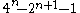. An equivalent formula is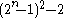. The first few Carol numbers are: −1, 7, 47
47 (number)
47 is the natural number following 46 and preceding 48.-In mathematics:Forty-seven is the fifteenth prime number, a safe prime, the thirteenth supersingular prime, and the sixth Lucas prime. Forty-seven is a highly cototient number...

, 223
223 (number)
223 is the natural number between 222 and 224. It is also a prime number.-In mathematics:223 is a long prime, a strong prime, a lucky prime and a sexy prime .223 is the fourth Carol number and the third to be prime....

, 959, 3967, 16127, 65023, 261119, 1046527 .

Carol numbers were first studied by Cletus Emmanuel, who named them after a friend, Carol G. Kirnon.

For n > 2, the binary representation of the n-th Carol number is n − 2 consecutive ones, a single zero in the middle, and n + 1 more consecutive ones, or to put it algebraically,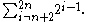So, for example, 47 is 101111 in binary, 223 is 11011111, etc. The difference between the 2n-th Mersenne number
Mersenne prime
In mathematics, a Mersenne number, named after Marin Mersenne , is a positive integer that is one less than a power of two: M_p=2^p-1.\,...

and the n-th Carol number is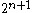. This gives yet another equivalent expression for Carol numbers,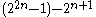. The difference between the n-th Kynea number and the n-th Carol number is the (n + 2)th power of two
Power of two
In mathematics, a power of two means a number of the form 2n where n is an integer, i.e. the result of exponentiation with as base the number two and as exponent the integer n....

.

Starting with 7, every third Carol number is a multiple of 7. Thus, for a Carol number to also be a prime number
Prime number
A prime number is a natural number greater than 1 that has no positive divisors other than 1 and itself. A natural number greater than 1 that is not a prime number is called a composite number. For example 5 is prime, as only 1 and 5 divide it, whereas 6 is composite, since it has the divisors 2...

, its index n cannot be of the form 3x + 2 for x > 0. The first few Carol numbers that are also prime are 7, 47, 223, 3967, 16127 (these are listed in Sloane's ). , the largest known Carol number that is also a prime is the Carol number for n = 253987, which has 152916 digits. It was found by Cletus Emmanuel in May 2007, using the programs MultiSieve and PrimeFormGW. It is the 40th Carol prime.

The 7th Carol number and 5th Carol prime, 16127, is also a prime when its digits are reversed, so it is the smallest Carol emirp
Emirp
An emirp is a prime number that results in a different prime when its digits are reversed. This definition excludes the related palindromic primes. Emirps are also called reversible primes....

. The 12th Carol number and 7th Carol prime, 16769023, is also a Carol emirp.